# Identifying Conic Sections: General Form & Standard Form

An error occurred trying to load this video.

Try refreshing the page, or contact customer support.

Coming up next: How to Use the Fundamental Counting Principle

### You're on a roll. Keep up the good work!

Replay
Your next lesson will play in 10 seconds
• 0:04 Conic Sections
• 2:31 Circle
• 3:15 Ellipse
• 3:43 Parabola
• 4:17 Hyperbola
• 4:59 Lesson Summary
Save Save

Want to watch this again later?

Timeline
Autoplay
Autoplay
Speed Speed Audio mode

#### Recommended Lessons and Courses for You

Lesson Transcript
Instructor: Yuanxin (Amy) Yang Alcocer

Amy has a master's degree in secondary education and has taught math at a public charter high school.

Did you know that if you slice a cone in different ways, you can make a circle, an ellipse, a parabola or a hyperbola? And, did you know that you can express all these shapes with just one general equation?

## Conic Sections

Imagine one of those bright orange traffic cones that you see on the road. Now, if you were to slice through that single cone at different angles, you'll see that you can end up with several different unique flat shapes. You get circles, ellipses, and parabolas. And if you glue two traffic cones tip to tip and slice through both, you'll get a hyperbola. The shapes that you get from slicing a cone are called conic sections.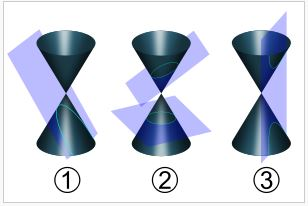In the picture above, the number one cone is the parabola. It looks like a u-curve. In number two, the bottom slice gives you a circle, a round shape where any point on the edge is the same distance to a central point. The top slice in number two is the ellipse, which is an elongated circle, or an oval. The slice in number three cuts through both cones straight down, and this gives you a hyperbola, two equal parabolas that look like mirror images of each other.

And of course, because this is math, you have formulas for all of these. This is the general formula for conic sections that covers all of your slice shapes: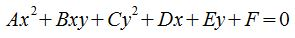In the formula, A, B, C, D, E, and F are all constants. This general form covers all four unique flat shapes. The values of these constants help to determine the shape. But you can't use the general form to determine the shape. You'll only know that by changing the general form to a standard form by completing the square for both the x and y variables.

To complete the square, rewrite the general form so all the x terms are together and all the y terms are together. Move the constant to the right side of the equation. Then complete the square for the x terms and the y terms, but remember to keep the equation balanced. Whatever you add or subtract on one side, you also have to do to the other. So, if you add 100 on the left side, you also have to add 100 on the right side. Then do some more math (divide everything by the constant on the right) so you end up with a one on the right side. You may want to change the denominators on the left side to be expressed as squares. Once you have the equation in standard form, you can determine whether it's a circle, ellipse, parabola, or hyperbola.

Here is an example of completing the square to convert from the general form to the standard form.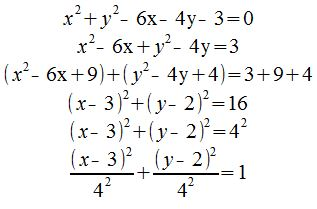Now that you've changed from general form to standard form, you can figure out what kind of shape it is.

## Circle

If it's a circle, then the standard form will follow this form: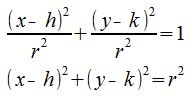The center of the circle is at the point (h, k) on the coordinate plane. The radius of the circle is r.

Because the circle has the radius as the denominator for both the x- and y-values, you can write the standard form for a circle in two ways, by either keeping the r term in the denominator on the left side or moving it to the right side.

Now, if you compare the equation you just changed (from the general form to the standard form) to the standard form for a circle, you see that it is a circle. It follows the standard form for a circle.

## Ellipse

The standard form for an ellipse follows this form: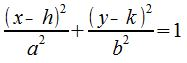To unlock this lesson you must be a Study.com Member.

### Register to view this lesson

Are you a student or a teacher?

#### See for yourself why 30 million people use Study.com

##### Become a Study.com member and start learning now.
Back
What teachers are saying about Study.com

### Earning College Credit

Did you know… We have over 200 college courses that prepare you to earn credit by exam that is accepted by over 1,500 colleges and universities. You can test out of the first two years of college and save thousands off your degree. Anyone can earn credit-by-exam regardless of age or education level.